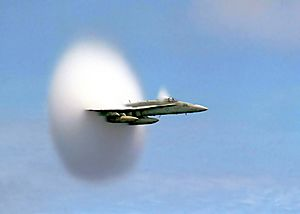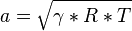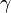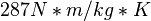# Speed of sound facts for kids

Kids Encyclopedia FactsU.S. Navy F/A-18 traveling near the speed of sound. The white halo consists of condensed water droplets formed by the sudden drop in air pressure behind the shock cone around the aircraft (see Prandtl-Glauert singularity).

The speed of sound is 1,235 kilometres (767 mi) per hour or 330 metres (1,083 ft) per second in dry air in room temperature. Sound moves faster through liquids and solids than air, since liquids and solids can be pushed together more. Sound cannot travel through a vacuum, which is a space without any air or matter. The speed of sound is affected by temperature. It travels slower at low temperatures, for example in the stratosphere.

You can calculate the speed of sound like this:$a = \sqrt{\gamma*R*T}$

Where:$\gamma$ is the ratio of specific heats (1.4 for air) R is the gas constant ($287 N*m/kg*K$ for air) T is temperature (in Kelvins)

The speed of sound is also known as Mach 1. Things that go faster are supersonic.

## Images for kidsSpeed of sound Facts for Kids. Kiddle Encyclopedia.